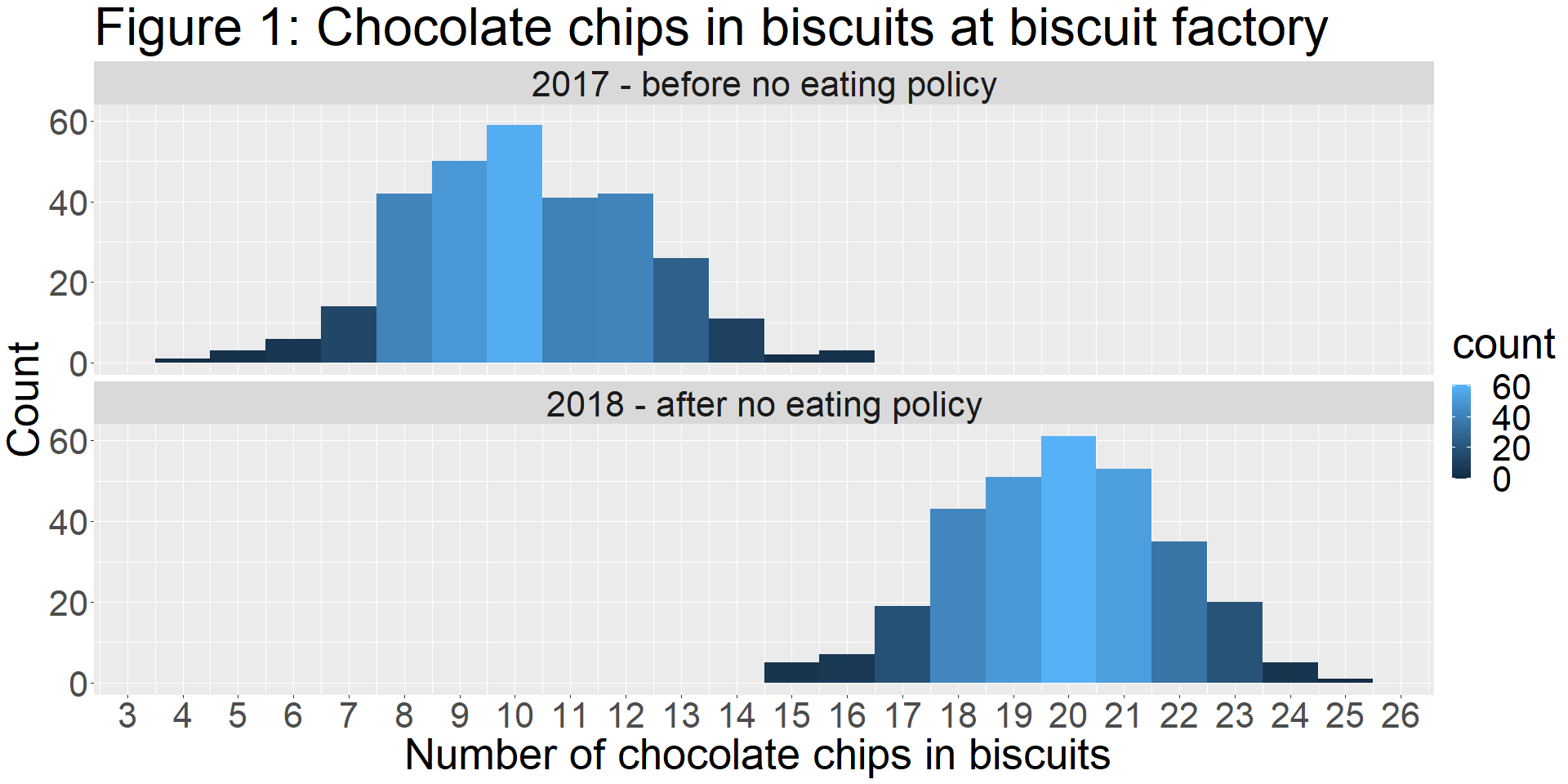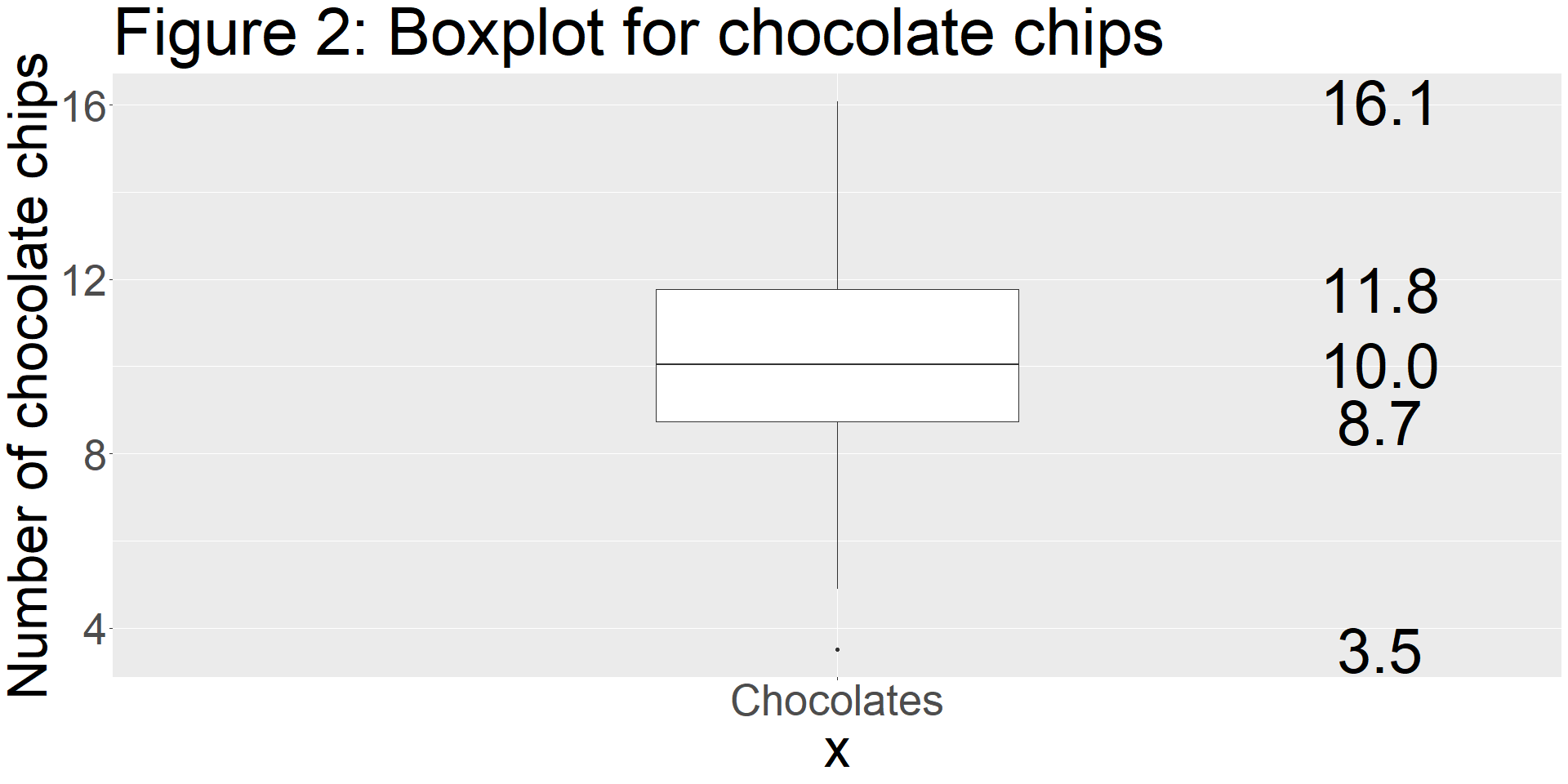# Overview

Statistical principles are used in most aspects of our daily lives; in choosing the lightest suitcase, the quietest street to live on, the biscuit with the most visible chocolate chips, the vaccine with a clinical trial that ticks the “most boxes”, the most commercially profitable business, and so on.

The way in which statistics is used and applied have be revolutionized over the past years. Machine learning techniques and sophisticated data science concepts/algorithms have expanded the scope for statistical applications.

The development of data science and machine learning concepts has also been accompanied by an advancement in the scope for data collection/capture. The avaiability of real time data capture, satellite images, and mobile apps has increased both the quantity and quality of data collection.

With sophisticated techniques for analysis and data collection there is a need for robust insight of core statistical concepts. Three early stages of statistical investigation are: construction of research hypotheses, data collection protocol, and data summarization/description.

Here, I begin with data summarization and focus on the measures of central tendency and measures of variation. I’ve used a fictional dataset, the chocolate biscuit dataset, to illustrate the concepts of central tendency and variation. This dataset is part of a “mystery series” which will be woven through the lectures so as to provide engaging applications to the statistical concepts presented.

In the Figure 1, I’ve used histograms to present a graphical overview of the number of chocolate chips found in bisucits within a chocolate biscuit factory before and after implementation of a policy. In the histograms for this example, the units on the x axis represent count categories of chocolate chips and the y axis represents the number of times (ie, the frequency) that a given count category is observed.

Simply put, Figure 1 illustrates the number of biscuits found with a given number of chocolate chips; before and after the new factory policy. Just for background, the policy requires that the employees refrain from eating inside the factory; this is because many biscuits have been discovered to be containing few chocolate chips and some biscuits seem to have been munched on.

In the histograms presented below, not only do we have a quick impression of the most frequently observed count of chocolate chips in biscuits (an indication of central tendency), but we also obtain an impression of the spread/distribution (an indication of variation) of the frequency of chocolate chip counts observed in the biscuits.# Measures of central tendency

Measures of central tendency enable us to provide a summary (“global view”) of our data; and preliminary indications of the nature of the variable/s involved.

### Mean

There are types of means; arithmetic, geometric and harmonic. For $$n$$ data points $$x_{i}$$, $$i=1:n$$, the arithmetic mean is calculated as dividing the sum of $$x_{i}$$ by $$n$$. This can be expressed as: $\frac{\sum_{i=1}^{n} x_{i}}{n}.$

Using our dataset, we can use the following R code to find the mean of chocolate chips before the new factory policy.

before = thebiscuits[which(thebiscuits$timeframe=="2017 - before no eating policy"),] round(mean(before$chocolate_chips),0)
##  10

### Median

To obtain the median, the data needs to be ordered from the smallest value to the largest. The data point in the “middle” of the ordered points is the median. In the case where the number of data points is an even number, the median is obtained by taking the mean of the two middle points (after the data is ordered).

Using our dataset, we can use the following R code to find the median of chocolate chips before the new factory policy.

before = thebiscuits[which(thebiscuits$timeframe=="2017 - before no eating policy"),] median(before$chocolate_chips)
##  10

### Mode

The mode is the data point which is occurs most frequently in the dataset.

Using our dataset, we can use the following R code to find the most frequently observed count of chocolate chips before the new factory policy. The frequency table in the output shows which count category was most frequently observed.

before = thebiscuits[which(thebiscuits$timeframe=="2017 - before no eating policy"),] thecounter = data.frame(summary(as.factor(before$chocolate_chips)))
colnames(thecounter)="Frequency"
thecounter$Category=rownames(thecounter) rownames(thecounter)=NULL kable(thecounter,caption="Table 1: Frequency table for chocolate chip count categories ")%>% kable_styling("striped", full_width = F) Table 1: Frequency table for chocolate chip count categories Frequency Category 1 4 3 5 6 6 14 7 42 8 50 9 59 10 41 11 42 12 26 13 11 14 2 15 3 16 In this example, the mean,median and mode were identical (the raw data was rounded for these calculations). In lecture two we will discuss the significance of this observation. # Measures of variation ### Range This measure of variation is commonly used, and many consider to be the most basic method. If $$x_{1}$$ and $$x_{2}$$ are the maximum and minimum values in a dataset, then the range is obtained by subtracting $$x_{2}$$ from $$x_{1}$$. ### Variance and Standard deviation The variance is obtained by finding the arithmetic mean of the set of $$(d_{i})^{2}$$, $$i=1:n$$, where $$d_{i}$$ is denoted as the difference between $$x_{i}$$ and the mean. The standard deviation is obtained by taking the square root of the variance. ##### Population variance It is important to note that when calculating the population variance $$\sigma^{2}$$, the number of data points $$n$$ is used as the denominator as in the following formula: $\sigma^{2} = \frac{\sum_{i=1}^{n} (d_{i})^{2}}{n}$ where $$d_{i}$$ is expressed as: $d_{i} = x_{i}-\mu$ and $$\mu$$ represents the population mean. ##### Sample variance When calculating the sample variance (where the dataset in question represents data sampled from the population), the following equation is used: $s^{2} = \frac{\sum_{i=1}^{n-1} (d_{i})^{2}}{n-1}$where $$d_{i}$$ is expressed as: $d_{i} = x_{i}-\bar{x}$ and $$\bar{x}$$ represents the sample mean. In the fictional chocolate biscuit example, the sample variance obtained for a sample of biscuits in a given shipment would be calculated differently from the variance calculated if every biscuit in that shipment was evaluated. ### Quartiles If the data points are ordered from the lowest to the highest value, the lower quartile, $$Q_{1}$$ denotes the data point below which 25% of the data lies. The second quartile, $$Q_{2}$$, which is also the median, refers to 50% of the data, and the third quartile,$$Q_{3}$$, 75%. The interquartile range, calculated by subtracting $$Q_{3}$$ from $$Q_{1}$$ is another useful measure of the spread of the data. In Figure 2 the three quartiles associated with the data on chocolate chips in the biscuit sample before the policy. Figure 2 is a boxplot which is one way in which the distribution of data can be presented. The plot is associated with five numbers. From top to bottom these are: the maximum observed value, the third quartile, the second quartile, the first quartile and the minimum observed value. thebiscuits = read.csv("C:/Users/glenn/Desktop/July 2021/May 2020/Lectures/data/cookie_second_recipe.csv") before = thebiscuits[which(thebiscuits$timeframe=="2017 - before no eating policy"),]
p<-ggplot(before, aes(y=chocolate_chips,x="Chocolates")) +
geom_boxplot(width=0.3)+
theme(text = element_text(size=50))+ylab("Number of chocolate chips")+
ggtitle("Figure 2: Boxplot for chocolate chips")+
stat_summary(geom="text", fun.y=quantile,
aes(label=sprintf("%1.1f", ..y..)),
position=position_nudge(x=0.45), size=20)
p# Conducting initial summary diagnostics in R

Finally, the inbuilt functions “head” and “summary” in R are useful starting points for investigating a variable of interest. Below, I’ve illustrated this with the biscuit dataset.

### Observing a “snapshot” of the dataset (subsetting only the data before the policy)

head(before)
##   Avg.choc.chips                      timeframe
## 1       7.479294 2017 - before no eating policy
## 2       9.480802 2017 - before no eating policy
## 3      12.694557 2017 - before no eating policy
## 4      10.569705 2017 - before no eating policy
## 5      10.508374 2017 - before no eating policy
## 6       7.865125 2017 - before no eating policy

### Obtaining a brief summary of the dataset (subsetting only the data before the policy)

summary(before)
##  Avg.choc.chips    timeframe
##  Min.   : 3.500   Length:300
##  1st Qu.: 8.738   Class :character
##  Median :10.050   Mode  :character
##  Mean   :10.174
##  3rd Qu.:11.773
##  Max.   :16.082